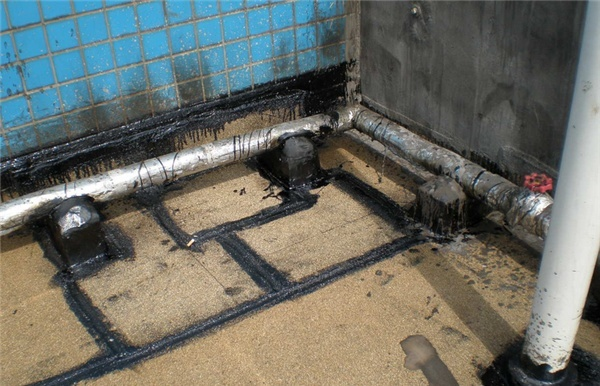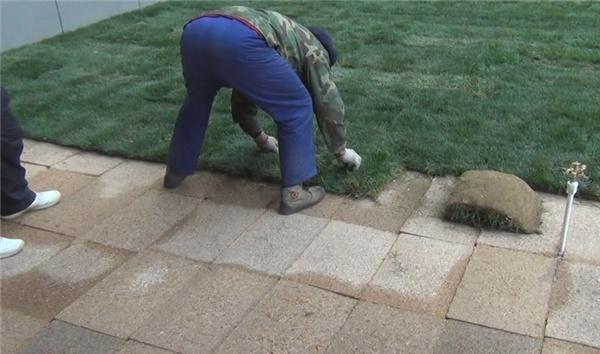|

# 屋顶花园防水怎么做 屋顶花园防水设计方法

随着生活水平的提高，现代人们更加注重生活品质，因此在屋顶设计花园成为最新的屋顶设计，为了防止以后出现漏水现象，我们需要配合进行防水施工，那屋顶花园防水怎么做？下面一起来看看吧！【屋顶花园防水怎么做】

1、不损伤原防水层

建造屋顶花园时必须进行二次防水处理，实施二次防水处理，最好先取掉屋顶的架空隔热层，取隔热层时，不得撬伤原防水层。取后要清扫、冲洗干净，以增强附着力。在一般情况下，不允许在已建成的屋顶防护水层上再穿孔洞与管线和预埋铁件与埋设支柱。因此，在新建房屋的屋顶上建屋顶花园时，应由园林设计部门提供屋顶花园的有关技术资料。

2、重视施工质量

屋顶花园防水层最好一次性全部施工完成，以免其中出现渗漏。防水层表面必须光洁平整，待施工完毕，刷2道防水涂料，以保证防水层的保护层设计与施工质量。要特别注意防水层的防腐蚀处理，防水层上的分格缝可用“一布四涂”盖缝，并选用耐腐蚀性能好的嵌缝油膏。不宜种植根系发达，对防水层有较强侵蚀作用的植物，如松、柏、榕树等。

3、注意材料质量

应选择高温不流淌、低温不碎裂、不宜老化、防水效果好的防水材料。刚性多层抹面水泥砂浆防水层宜采用标号不低于原325#的普通硅酸盐水泥和膨胀水泥，亦可采用矿渣硅酸盐水泥；砂采用粒径1~3mm粗砂，要求砂料坚硬、粗糙、洁净；水泥浆和水泥砂浆的配合比应根据防水要求、原材料性能和施工方法确定，施工时必须严格掌握。目前一些建筑物也有柔性防水层的，屋顶花园中常有“三毡四油”或“二毡三油”，再结合聚氯乙烯胶泥或聚氯乙烯涂料处理。近年来，一些新型防水材料也开始投入使用，已投入屋顶施工的有三元乙丙卷材，使用效果不错。国外还有尝试用中空类的泡沫塑料制品作为绿化土层与屋顶之间的良好排水层和填充物，以减轻自重。

4、做防水实验

做完二次防水处理后就要检查一下防水性。首先封闭出水口，再灌水，进行96小时的严格闭水试验。闭水试验中，要仔细观察房间的渗漏情况，有的房屋连续闭水3天不漏，第四天才开始渗漏。若能保证96小时不漏，说明屋面花园防水效果好。

5、检查排水系统

防水层是保证屋顶不漏的关键技术问题，但是还必须处理好屋顶花园的排水系统。在屋顶园林工程中，种植池、水池和道路场地施工时，应遵照原屋顶排水系统，进行规划设计，不应封堵、隔绝或改变原排水口和坡度。特别是大型种植池排水层下的排水管道，要与屋顶排水口配合，注意相关的标准差，使种植池排水层下的排水管道，要与屋顶排水口配合，注意相关的标高差，使种植池内的多余灌水能顺畅排出。【屋顶花园防水施工注意事项】

1、做防水实验和保证良好的排水系统

建造屋顶花园，必须进行二次防水处理。首先，要检查原有的防水性能：封闭出水口，再灌水，进行96小时（4天4夜）的严格闭水试验。闭水试验中，要仔细观察房间的渗漏情况，有的房屋连续闭水3天不漏，第四天才开始渗漏。若能保证96h不漏，说明屋面防水效果好。这种防水效果，也只适用于非屋顶花园的情况。防水层是保证屋顶不漏的关键技术问题，但屋顶防水和排水是一个两个方面，因此还必须处理好屋顶的排水系统。在屋顶园林工程中，种植池、水池和道路场地施工时，应遵照原屋顶排水系统，进行规划设计，不应封堵、隔绝或改变原排水口和坡度。特别是大型种植池排水层下的排水管道，要与屋顶排水口配合，注意相关的标准差，使种植池排水层下的排水管道，要与屋顶排水口配合，注意相关的标高差，使种植池内的多余灌水能顺畅排出。

2、不损伤原防水层

实施二次防水处理，最好先取掉屋顶的架空隔热层，取隔热层时，不得撬伤原防水层。取后要清扫、冲洗干净，以增强附着力。在一般情况下，不允许在已建成的屋顶防护水层上再穿孔洞与管线和预埋铁件与埋设支柱。因此，在新建房屋的屋顶上建屋顶花园时，应由园林设计部门提供屋顶花园的有关技术资料。如将欲留孔洞和欲埋件等资料提供给结构设计单位，并由他们将有关要求反映到建筑结构的施工图中，以便建筑施工中实现屋顶花园的各项技术要求。如果在旧建筑物上增建屋顶花园，无论是那种做法的屋面防水层，均不得在屋顶上穿洞打孔、埋设铁件和支柱。即使一般设备装置也不能在屋顶上“生根”，只能采取其他措施使他们“浮摆”在屋面上。

3、重视防水层的施工质量

目前屋顶花园的防水处理方法主要有刚、柔之分，各有特点。由于蛭石栽培对屋盖有很好的养护作用，此时屋顶防水最好采用刚性防水。宜先做涂膜防水层，再做刚性防水层，其做法可参照标准设计的构造详图。刚性防水层主要是屋面板上铺50㎜厚细石混凝土，内放双向钢筋网片1层（这种做法即成整筑层），所用混凝土中可加入适量微膨胀剂、减水剂、防水剂等，以提高其其抗裂、抗渗性能。这种防水层比较坚硬，能防止根系发达的乔灌木穿透，起到保护屋顶的作用，而且使整个屋顶有较好的整体性，不宜产生裂缝，使用寿命也较长，比柔性卷材防水层更适合建造屋顶花园。屋面四周应设置砖砌挡墙，挡墙下部设泄水孔和天沟。当种植屋面为柔性防水层时，上面还应设置1层刚性保护层。也就是说，屋面可以采用1道或多道（复合）防水设防，但最上面一道应为刚性防水层，屋面泛水的防水层高度应高出溢水口100㎜。【屋顶花园防水施工工艺】

一、前期准备

1、首先要将屋面打扫干净平整，如果有什么浮尘或者是屋面的油污脱膜剂松动等都要及时处理；

2、发现屋面有较大的孔洞以及裂缝的话就要及时用水泥砂浆进行填补平整；

3、对屋面浇水进行湿润，但是要切忌屋面积水，有积水会影响后期的施工；

4、屋面的伸缩缝需要用密封胶进行填补，密封胶质量的选择一定要优质，不会产生什么问题。

二、施工工艺

step1：将K11防水浆料添加剂倒入干净的容器中进行搅拌，一边搅拌一边缓慢的加入粉料，成没有分团的胶浆即可；

step2：使用毛刷或者是滚筒沾取搅拌好的胶浆在屋面上进行涂刷，涂刷的时候一定要保持均匀；

step3：第一层涂刷完毕后隔3个小时左右再进行第二层涂刷，在做之前记得先在第一层洒上少许水进行湿润再施工；

step4：涂刷完毕后在24小时以内禁止在上面践踏，遇到阴雨天气的时候要覆盖上一层保护膜，这样才能够有效的进行保护；

step5：浆料完全干透后就可以在屋顶进行填土种花了，这样屋顶花园防水就做好了。

三、工艺说明

这种屋顶花园防水施工工艺相对来说是比较简单的一种方式，主要采取的是K11防水浆料进行防水，施工起来也非常的方便快捷，而且防水层还具有非常好的柔韧性，黏结性能也非常的好，而且还具有非常好的耐候性，也不再需要其它特别的保护层进行保护，可见这种屋顶花园防水真的是非常不错的。如果你觉得这种方式非常符合你的需求的话，那么就尝试着做一下吧。

`声明：本文由入驻焦点开放平台的作者撰写，除焦点官方账号外，观点仅代表作者本人，不代表焦点立场错误信息举报电话： 400-099-0099，邮箱：jubao@vip.sohu.com，或点此进行意见反馈，或点此进行举报投诉。`A B C D E F G H J K L M N P Q R S T W X Y Z
A - B - C - D - E
• A
• 鞍山
• 安庆
• 安阳
• 安顺
• 安康
• 澳门
• B
• 北京
• 保定
• 包头
• 巴彦淖尔
• 本溪
• 蚌埠
• 亳州
• 滨州
• 北海
• 百色
• 巴中
• 毕节
• 保山
• 宝鸡
• 白银
• 巴州
• C
• 承德
• 沧州
• 长治
• 赤峰
• 朝阳
• 长春
• 常州
• 滁州
• 池州
• 长沙
• 常德
• 郴州
• 潮州
• 崇左
• 重庆
• 成都
• 楚雄
• 昌都
• 慈溪
• 常熟
• D
• 大同
• 大连
• 丹东
• 大庆
• 东营
• 德州
• 东莞
• 德阳
• 达州
• 大理
• 德宏
• 定西
• 儋州
• 东平
• E
• 鄂尔多斯
• 鄂州
• 恩施
F - G - H - I - J
• F
• 抚顺
• 阜新
• 阜阳
• 福州
• 抚州
• 佛山
• 防城港
• G
• 赣州
• 广州
• 桂林
• 贵港
• 广元
• 广安
• 贵阳
• 固原
• H
• 邯郸
• 衡水
• 呼和浩特
• 呼伦贝尔
• 葫芦岛
• 哈尔滨
• 黑河
• 淮安
• 杭州
• 湖州
• 合肥
• 淮南
• 淮北
• 黄山
• 菏泽
• 鹤壁
• 黄石
• 黄冈
• 衡阳
• 怀化
• 惠州
• 河源
• 贺州
• 河池
• 海口
• 红河
• 汉中
• 海东
• I
• J
• 晋中
• 锦州
• 吉林
• 鸡西
• 佳木斯
• 嘉兴
• 金华
• 景德镇
• 九江
• 吉安
• 济南
• 济宁
• 焦作
• 荆门
• 荆州
• 江门
• 揭阳
• 金昌
• 酒泉
• 嘉峪关
K - L - M - N - P
• K
• 开封
• 昆明
• 昆山
• L
• 廊坊
• 临汾
• 辽阳
• 连云港
• 丽水
• 六安
• 龙岩
• 莱芜
• 临沂
• 聊城
• 洛阳
• 漯河
• 娄底
• 柳州
• 来宾
• 泸州
• 乐山
• 六盘水
• 丽江
• 临沧
• 拉萨
• 林芝
• 兰州
• 陇南
• M
• 牡丹江
• 马鞍山
• 茂名
• 梅州
• 绵阳
• 眉山
• N
• 南京
• 南通
• 宁波
• 南平
• 宁德
• 南昌
• 南阳
• 南宁
• 内江
• 南充
• P
• 盘锦
• 莆田
• 平顶山
• 濮阳
• 攀枝花
• 普洱
• 平凉
Q - R - S - T - W
• Q
• 秦皇岛
• 齐齐哈尔
• 衢州
• 泉州
• 青岛
• 清远
• 钦州
• 黔南
• 曲靖
• 庆阳
• R
• 日照
• 日喀则
• S
• 石家庄
• 沈阳
• 双鸭山
• 绥化
• 上海
• 苏州
• 宿迁
• 绍兴
• 宿州
• 三明
• 上饶
• 三门峡
• 商丘
• 十堰
• 随州
• 邵阳
• 韶关
• 深圳
• 汕头
• 汕尾
• 三亚
• 三沙
• 遂宁
• 山南
• 商洛
• 石嘴山
• T
• 天津
• 唐山
• 太原
• 通辽
• 铁岭
• 泰州
• 台州
• 铜陵
• 泰安
• 铜仁
• 铜川
• 天水
• 天门
• W
• 乌海
• 乌兰察布
• 无锡
• 温州
• 芜湖
• 潍坊
• 威海
• 武汉
• 梧州
• 渭南
• 武威
• 吴忠
• 乌鲁木齐
X - Y - Z
• X
• 邢台
• 徐州
• 宣城
• 厦门
• 新乡
• 许昌
• 信阳
• 襄阳
• 孝感
• 咸宁
• 湘潭
• 湘西
• 西双版纳
• 西安
• 咸阳
• 西宁
• 仙桃
• 西昌
• Y
• 运城
• 营口
• 盐城
• 扬州
• 鹰潭
• 宜春
• 烟台
• 宜昌
• 岳阳
• 益阳
• 永州
• 阳江
• 云浮
• 玉林
• 宜宾
• 雅安
• 玉溪
• 延安
• 榆林
• 银川
• Z
• 张家口
• 镇江
• 舟山
• 漳州
• 淄博
• 枣庄
• 郑州
• 周口
• 驻马店
• 株洲
• 张家界
• 珠海
• 湛江
• 肇庆
• 中山
• 自贡
• 资阳
• 遵义
• 昭通
• 张掖
• 中卫

1室1厅1厨1卫1阳台

1
2
3
4
5

0
1
2

1

1

0
1
2
3报名成功，资料已提交审核A B C D E F G H J K L M N P Q R S T W X Y Z
A - B - C - D - E
• A
• 鞍山
• 安庆
• 安阳
• 安顺
• 安康
• 澳门
• B
• 北京
• 保定
• 包头
• 巴彦淖尔
• 本溪
• 蚌埠
• 亳州
• 滨州
• 北海
• 百色
• 巴中
• 毕节
• 保山
• 宝鸡
• 白银
• 巴州
• C
• 承德
• 沧州
• 长治
• 赤峰
• 朝阳
• 长春
• 常州
• 滁州
• 池州
• 长沙
• 常德
• 郴州
• 潮州
• 崇左
• 重庆
• 成都
• 楚雄
• 昌都
• 慈溪
• 常熟
• D
• 大同
• 大连
• 丹东
• 大庆
• 东营
• 德州
• 东莞
• 德阳
• 达州
• 大理
• 德宏
• 定西
• 儋州
• 东平
• E
• 鄂尔多斯
• 鄂州
• 恩施
F - G - H - I - J
• F
• 抚顺
• 阜新
• 阜阳
• 福州
• 抚州
• 佛山
• 防城港
• G
• 赣州
• 广州
• 桂林
• 贵港
• 广元
• 广安
• 贵阳
• 固原
• H
• 邯郸
• 衡水
• 呼和浩特
• 呼伦贝尔
• 葫芦岛
• 哈尔滨
• 黑河
• 淮安
• 杭州
• 湖州
• 合肥
• 淮南
• 淮北
• 黄山
• 菏泽
• 鹤壁
• 黄石
• 黄冈
• 衡阳
• 怀化
• 惠州
• 河源
• 贺州
• 河池
• 海口
• 红河
• 汉中
• 海东
• I
• J
• 晋中
• 锦州
• 吉林
• 鸡西
• 佳木斯
• 嘉兴
• 金华
• 景德镇
• 九江
• 吉安
• 济南
• 济宁
• 焦作
• 荆门
• 荆州
• 江门
• 揭阳
• 金昌
• 酒泉
• 嘉峪关
K - L - M - N - P
• K
• 开封
• 昆明
• 昆山
• L
• 廊坊
• 临汾
• 辽阳
• 连云港
• 丽水
• 六安
• 龙岩
• 莱芜
• 临沂
• 聊城
• 洛阳
• 漯河
• 娄底
• 柳州
• 来宾
• 泸州
• 乐山
• 六盘水
• 丽江
• 临沧
• 拉萨
• 林芝
• 兰州
• 陇南
• M
• 牡丹江
• 马鞍山
• 茂名
• 梅州
• 绵阳
• 眉山
• N
• 南京
• 南通
• 宁波
• 南平
• 宁德
• 南昌
• 南阳
• 南宁
• 内江
• 南充
• P
• 盘锦
• 莆田
• 平顶山
• 濮阳
• 攀枝花
• 普洱
• 平凉
Q - R - S - T - W
• Q
• 秦皇岛
• 齐齐哈尔
• 衢州
• 泉州
• 青岛
• 清远
• 钦州
• 黔南
• 曲靖
• 庆阳
• R
• 日照
• 日喀则
• S
• 石家庄
• 沈阳
• 双鸭山
• 绥化
• 上海
• 苏州
• 宿迁
• 绍兴
• 宿州
• 三明
• 上饶
• 三门峡
• 商丘
• 十堰
• 随州
• 邵阳
• 韶关
• 深圳
• 汕头
• 汕尾
• 三亚
• 三沙
• 遂宁
• 山南
• 商洛
• 石嘴山
• T
• 天津
• 唐山
• 太原
• 通辽
• 铁岭
• 泰州
• 台州
• 铜陵
• 泰安
• 铜仁
• 铜川
• 天水
• 天门
• W
• 乌海
• 乌兰察布
• 无锡
• 温州
• 芜湖
• 潍坊
• 威海
• 武汉
• 梧州
• 渭南
• 武威
• 吴忠
• 乌鲁木齐
X - Y - Z
• X
• 邢台
• 徐州
• 宣城
• 厦门
• 新乡
• 许昌
• 信阳
• 襄阳
• 孝感
• 咸宁
• 湘潭
• 湘西
• 西双版纳
• 西安
• 咸阳
• 西宁
• 仙桃
• 西昌
• Y
• 运城
• 营口
• 盐城
• 扬州
• 鹰潭
• 宜春
• 烟台
• 宜昌
• 岳阳
• 益阳
• 永州
• 阳江
• 云浮
• 玉林
• 宜宾
• 雅安
• 玉溪
• 延安
• 榆林
• 银川
• Z
• 张家口
• 镇江
• 舟山
• 漳州
• 淄博
• 枣庄
• 郑州
• 周口
• 驻马店
• 株洲
• 张家界
• 珠海
• 湛江
• 肇庆
• 中山
• 自贡
• 资阳
• 遵义
• 昭通
• 张掖
• 中卫• 手机• 分享
• 设计
免费设计
• 计算器
装修计算器
• 入驻
合作入驻
• 联系
联系我们
• 置顶
返回顶部